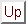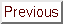Next: Hock and Schittkowski set Up: Numerical Results of CONDOR. Previous: Numerical Results of CONDOR.   Contents

# Random objective functions

We will use for the tests, the following objective function: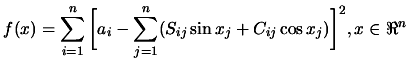(7.1)

The way of generating the parameters ofis taken from [RP63], and is as follows. The elements of thematricesand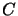are random integers from the interval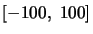, and a vectoris chosen whose components are random numbers from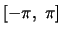. Then, the parameters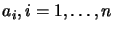are defined by the equation, and the starting vector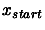is formed by adding random perturbations of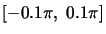to the components of. All distributions of random numbers are uniform. There are two remarks to do on this objective function:
• Because the number of terms in the sum of squares is equals to the number of variables, it happens often that the Hessian matrix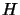is ill-conditioned around.
• Becauseis periodic, it has many saddle points and maxima.
Using this test function, it is possible to cover every kind of problems, (from the easiest one to the most difficult one).
We will compare the CONDOR algorithm with an older algorithm: ''CFSQP''. CFSQP uses line-search techniques. In CFSQP, the Hessian matrix of the function is reconstructed using aupdate, the gradient is obtained by finite-differences.
Parameters of CONDOR: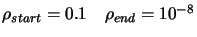.
Parameters of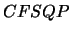:. The algorithm stops when the step size is smaller than.
Recalling that, we will say that we have a success when the value of the objective function at the final point of the optimization algorithm is lower then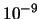.
We obtain the following results, after 100 runs of both algorithms:
 Mean number of Mean best value of Dimensionfunction evaluations Number of success the objective function of the space CONDOR CFSQP CONDOR CFSQP CONDOR CFSQP 3 44.96 246.19 100 46 3.060873e-017 5.787425e-011 5 99.17 443.66 99 27 5.193561e-016 8.383238e-011 10 411.17 991.43 100 14 1.686634e-015 1.299753e-010 20 1486.100000 -- 100 -- 3.379322e-016 --

We can now give an example of execution of the algorithm to illustrate the discussion of Section 6.2:
 Rosenbrock's function () function evaluations Best Value So Far33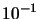88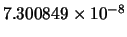91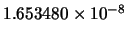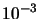9497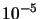100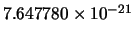101103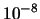With the Rosenbrock's function=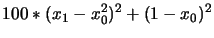We will use the same choice of parameters (for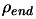and) as before. The starting point is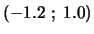.
As you can see, the number of evaluations performed whenis reduced is far inferior to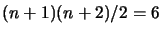.Next: Hock and Schittkowski set Up: Numerical Results of CONDOR. Previous: Numerical Results of CONDOR.   Contents
Frank Vanden Berghen 2004-04-19